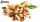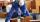# Together

Grandfather, father, and son are carpenters. They decided to make a wardrobe.
Father produces it for 12 hours, his son for 15 hours, and grandfather for 10 hours.
How long will they make it together?

Correct result:

x =  4 h

#### Solution:

x•(1/12+1/15+1/10)=1

15x = 60

x = 4

Our simple equation calculator calculates it.We would be pleased if you find an error in the word problem, spelling mistakes, or inaccuracies and send it to us. Thank you!Tips to related online calculators
Need help calculate sum, simplify or multiply fractions? Try our fraction calculator.
Do you have a linear equation or system of equations and looking for its solution? Or do you have quadratic equation?

## Next similar math problems:

• Unknown numberIdentify unknown number which 1/5 is 40 greater than one tenth of that number.
• Hectoliters of waterThe pool has a total of 126 hectoliters of water. The first pump draws 2.1 liters of water per second. A second pump pumps 3.5 liters of water per second. How long will it take both pumps to drain four-fifths of the water at the same time?
• Simple equationSolve for x: 3(x + 2) = x - 18
• One thirdIf 3/5 is 360, how much is 1/3?
• Unknown numberI think the number - its sixth is 3 smaller than its third.
• Simple equation 6Solve equation with one variable: X/2+X/3+X/4=X+4
• PearsThere were pears in the basket, I took two-fifths of them, and left six in the basket. How many pears did I take?
• FractionsThree-quarters of an unknown number are 4/5. What is 5/6 of this unknown number?
• UN 1If we add to an unknown number his quarter, we get 210. Identify unknown number.
• Bag of peanutsJoe eat 1/3 of a bag of peanuts, mark eat 1/4 of the remaining in the bag of peanuts, Alvin eat 1/2 of the remaining bag of peanuts, peter eat 10 peanuts, there are 71 peanuts left. Hon many peanuts were in the bags?
• Equation with xSolve the following equation: 2x- (8x + 1) - (x + 2) / 5 = 9
• Eq-fracSolve the following equation with fractions: h + 1/3 =5/3
• EqnSolve equation with fractions: 2x/3-50=40+x/4
• Cleaning windowsCleaning company has to wash all the windows of the school. The first day washes one-sixth of the windows of the school, the next day three more windows than the first day and the remaining 18 windows washes on the third day. Calculate how many windows ha
• Fraction + eqSolve following simple equation with fractions: -5/6(8+5b) = 75 + 5/3b
• Equation with fractionsSolve equation: ? It is equation with fractions.
• Bricklayers8 bricklayers build a house for 630 days. How many bricklayers have to add after 150 days, so then the whole building completed in (next) 320 days?